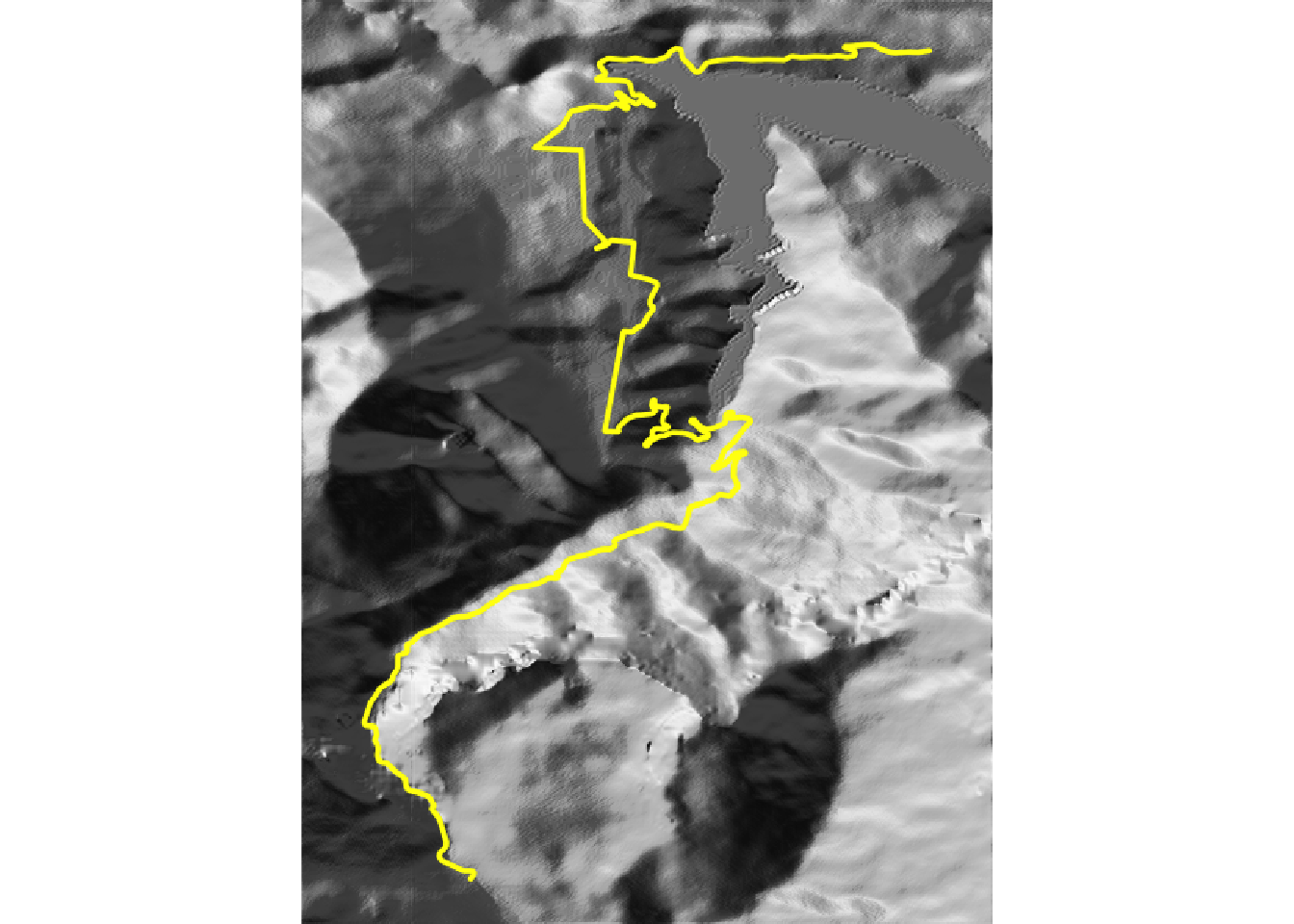# 13 Rendering 3D `rayshader` Stage Route Maps

Having introduced interactive 3d `rayshader` models in the previous chapter, let’s now explore how we can generate interactive three dimensional models of our stage maps.

## 13.1 Load in Base Data

As ever, let’s load in our stage data:

``````library(tidyr)

geojson_filename = 'montecarlo_2021.geojson'
``````## Reading layer `montecarlo_2021' from data source `/Users/tonyhirst/Documents/GitHub/visualising-rally-stages/montecarlo_2021.geojson' using driver `GeoJSON'
## Simple feature collection with 9 features and 2 fields
## geometry type:  LINESTRING
## dimension:      XY
## bbox:           xmin: 5.243488 ymin: 43.87633 xmax: 6.951953 ymax: 44.81973
## geographic CRS: WGS 84``````
``stage_bbox = sf::st_bbox(geojson_sf)``

We have already seen how we can overlay an elevation model raster on a leaflet map, as well as rendering two dimensional topographic models using the `raytracer` package. Generating 3D, rather than 2D, maps follows exactly the same steps as the 2D view apart from the final rendering step.

Recall that the `raytracer` package itself works with a matrix of raster values. Having saved the download raster to a tif file, we can load the data back in from and convert it to a matrix using the `rayshader::raster_to_matrix()` function:

``````library(rayshader)
library(raster)

stage_tif = "buffered_stage_elevation.tif"

# Load in the previously saved image raster
elev_img = raster(stage_tif)

# Get the natural zscale
auto_zscale = geoviz::raster_zscale(elev_img)

# Note we can pass in a file name or a raster object
elmat = raster_to_matrix(stage_tif) ``````

Just as a reminder, here’s a quick review of what the 2D maps look like:

``````# Use the raster extent for the plots
stage_extent = extent(elev_img)

yellow_route = generate_line_overlay(geojson_sf[1,],
extent = stage_extent,
heightmap = elmat,
linewidth = 5, color="yellow")

mapped_route_yellow = elmat %>%
sphere_shade(sunangle = -45, texture = "bw") %>%

mapped_route_yellow %>%
plot_map()``````The 2D plots can be quite pretty, but we can also bring them even more alive as 3D rendered plots.

## 13.2 Setting Up 3D Embedded Plots

Recall that to embed WebGL interactive models using `rgl::rglwidget()`, we need to fettle some settings first:

``````options(rgl.useNULL = TRUE,
rgl.printRglwidget = TRUE)``````

## 13.3 Rendering a Simple Stage Route Model

We can render our 2D route model simply by passing it to the `rayshader::plot_3d()` function, along with the elevation model.

If we set the `zscale` parameter to the auto_zscale determined previously as `auto_zscale = geoviz::raster_zscale(elev_img)`, the relief is rendered using the “real” scaling that keeps the height of elevated areas in equal proportion to the scale used by the x and y scale values:

``````rgl::clear3d()

mapped_route_yellow %>%
plot_3d(elmat, zscale=auto_zscale)

rgl::rglwidget()``````